# [3.NF.3d-1.0] Compare Similar Fractions - Common Core Standard - Practice Problem

##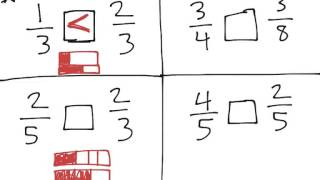By Freckle education

Compare two fractions with the same numerator or the same denominator by reasoning about their size# Comparing Fractions (3.NF.3D)

##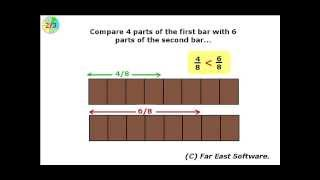By Far East Software

Compare two fractions with the same numerator or the same denominator by reasoning about their size# [3.NF.3d-1.1] Compare Similar Fractions - Common Core Standard

##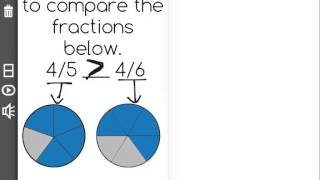By Freckle education

Compare two fractions with the same numerator by reasoning about their size# More on equivalent fractions | Fractions | 4th grade | Khan Academy

##By Khan Academy

Important: if you multiply a fraction's numerator and the denominator by the same number, you'll end up with an equivalent fraction. Got it? Maybe? No problem, we'll help!# Multiplying Integers | MathHelp.com

##By MathHelp.com

This lesson covers comparing fractions. Students learn to compare fractions with the same denominator, which are called like fractions, by comparing the numerators. For example, to compare 7/9 and 4/9, note that 7 is greater than 4, so 7/9 is greater than 4/9. Students also learn to compare fractions with the different denominators, which are called unlike fractions, by first finding a common denominator, then comparing the numerators. For example, to compare 1/2 and 1/3, first find a common denominator, or the Least Common Multiple of 2 and 3, which is 6. To get 6 in the denominator of 1/2, multiply the numerator and denominator by 3, to get 3/6. To get 6 in the denominator of 1/3, multiply the numerator and denominator by 2, to get 2/6. Next, compare 3/6 and 2/6. Note that 3 is greater than 2, so 3/6 is greater than 2/6, which means that 1/2 is greater than 1/3.# [4.NF.2-1.0] Compare Dissimilar Fractions - Common Core Standard

##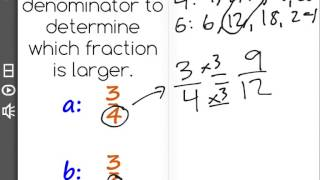By Freckle by Renaissance

Compare two fractions with different numerators and different denominators, e.g., by creating common denominators or numerators, or by comparing to a benchmark fraction such as 1/2. Recognize that comparisons are valid only when the two fractions refer to the same whole. Record the results of comparisons with symbols greater than , = , or less than , and justify the conclusions, e.g., by using a visual fraction model. Front Row is a free, adaptive, Common Core aligned math program for teachers and students in kindergarten through eighth grade. Front Row allows students to practice math at their own pace - learning advanced concepts when they're ready and receiving remediation when they struggle. Front Row provides teachers with access to a detailed data dashboard and weekly email reports that show which standards are causing students difficulty, what small groups can be formed for interventions, and how their students are progressing in math.# Lowest Common Denominator | Subtracting Fractions | MathHelp.com

##By MathHelp.com# Multiplying Fractions | MathHelp.com

##By MathHelp.com# Equivalent Fractions How To Teach Common Core Math to 3rd or 4th Graders

##By Promoting Success##By MathHelp.com# Comparing Fractions | MathHelp.com

##By MathHelp.com

MathHelp.com offers comprehensive College Algebra help with a teacher. Are you stuck in a College Algebra class with a bad teacher and a huge classroom? Take the matter into your own hands with our custom College Algebra course. You will get through college math in no time!# Dividing Fractions | MathHelp.com

##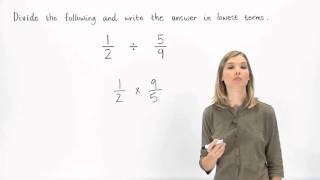By MathHelp.com# Exponents | MathHelp.com

##By MathHelp.com# Adding and Subtracting Fractions with the Same Denominator

##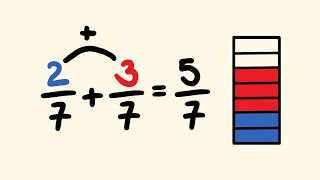By tecmath# Fraction Word Problems | MathHelp.com

##By MathHelp.com# Dividing Mixed Numbers | MathHelp.com

##By MathHelp.com# Simplifying Rational Expressions | MathHelp.com

##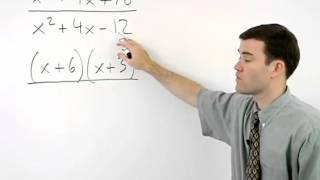By MathHelp.com# 01 - What are Equivalent Fractions? - (Calculate & Find Equivalent Fractions) - Part 1

##By Math and Science

Quality Math And Science Videos that feature step-by-step example problems!# Learn to Subtract Fractions (Adding & Subtracting Fractions) - Part 1 

##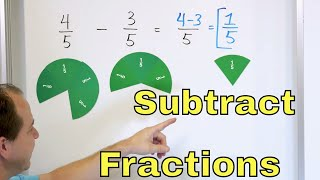By Math and Science# Types Of Fractions - Review | Maths For Grade 5 | Periwinkle

##By Lumos Learning

Types Of Fractions - Review | Maths For Grade 5 | Periwinkle